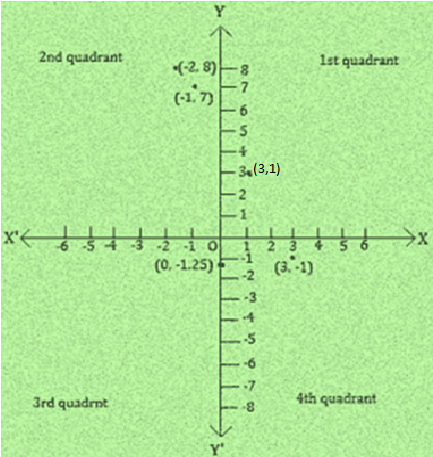Newbie

# Plot the points (x, y) given in the following table on the plane, choosing suitable units of distance on the axes.Q.2

• 0

Find the best solution of chapter coordinate geometry of ncert class 9th give me the best soluiton of exercise 3.3 question 2 . How i solve this question in a easiest and simplest way . Plot the points (x, y) given in the following table on the plane, choosing suitable units of distance on the axes

Share

1. Solution:

The points to plotted on the (x, y) are:

i. (-2, 8)

ii. (-1, 7)

iii. (0, -1.25)

iv. (1, 3)

v. (3, -1)

On the graph mark X-axis and Y-axis. Mark the meeting point as O.

Now, Let 1 unit = 1 cmi. (-2, 8): II- Quadrant, Meeting point of the imaginary lines that starts from 2 units to the left of origin O and from 8 units above the origin O

ii. (-1, 7): II- Quadrant, Meeting point of the imaginary lines that starts from 1 units to the left of origin O and from 7 units above the origin O

iii. (0, -1.25): On the x-axis, 1.25 units to the left of origin O

iv. (1, 3): I- Quadrant, Meeting point of the imaginary lines that starts from 1 units to the right of origin O and from 3 units above the origin O

v. (3, -1): IV- Quadrant, Meeting point of the imaginary lines that starts from 3 units to the right of origin O and from 1 units below the origin O

• 0